# KSEEB Solutions for Class 9 Maths Chapter 13 Surface Area and Volumes Ex 13.4

In this chapter, we provide KSEEB SSLC Class 9 Maths Chapter 13 Surface Area and Volumes Ex 13.4 for English medium students, Which will very helpful for every student in their exams. Students can download the latest KSEEB SSLC Class 9 Maths Chapter 13 Surface Area and Volumes Ex 13.4 pdf, free KSEEB SSLC Class 9 Maths Chapter 13 Surface Area and Volumes Ex 13.4 pdf download. Now you will get step by step solution to each question.

## Karnataka Board Class 9 Maths Chapter 13 Surface Area and Volumes Ex 13.4

(Assume π = 227, unless stated otherwise)

Question 1.
Find the surface area of a sphere of radius :
(i) 10.5 cm
(ii) 5.6 cm
(iii) 14 cm.
Solution: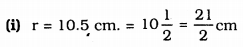Surface area of the sphere = 4πr2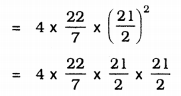= 22 × 3 × 21
= 1386 cm2.

(ii) r = 5.6 cm. = 5610cm.
Surface area of the sphere = 4πr2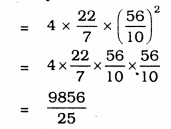= 394.24 cm2.

(iii) r = 14 cm.
Surface area of the sphere = 4πr2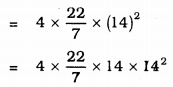= 88 × 28
= 2464 cm2.

Question 2.
Find the surface area of a sphere of diameter :
(i) 14 cm
(ii) 21 cm
(iii) 3.5 m.
Solution:
(i) Diameter, d = 14 cm.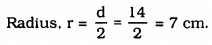Surface area of a sphere = 4πr2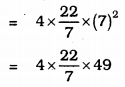= 616 cm2.
(ii) Diameter, d = 21 cm.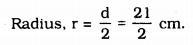Surface area of a sphere = 4πr2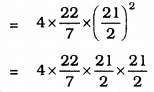= 1386 cm2.
(iii) Diameter, d = 3.5 m. = 72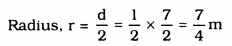Surface area of a sphere = 4πr2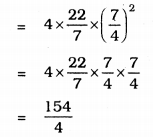= 38.5 m2.

Question 3.
Find the total surface area of a hemisphere of radius 10 cm.
(Use π = 3.14 )
Solution:
r = 10 cm.
Total surface area of a hemisphere = 3πr2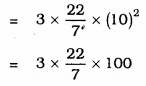= 942 cm2.

Question 4.
The radius of a spherical balloon increases from 7 cm to 14 cm as air is being pumped into it. Find the ratio of surface areas of the balloon in the two cases.
Solution:
Radius of spherical balloons be r1 and r2, r1 = 7 cm. and r2 = 14 cm.
Ratio of surface area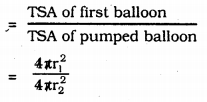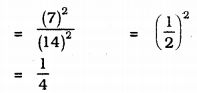∴ Ratio of surface areas of the balloons = 1 : 4.

Question 5.
A hemispherical bowl made of brass has an inner diameter of 10.5 cm. Find the cost of tin-plating it on the inside at the rate of Rs. 16 per 100 cm2.
Solution:
Inner diameter of hemispherical bowl = 10.5 cm.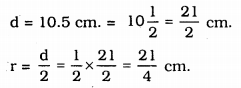Curved surface area of the bowl = 2πr2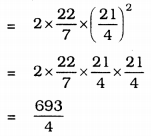= 173.25 cm2.
Cost of tin-plating for 100 cm2 is Rs. 16.
Cost of tin-plating for 173.25 sq.cm. …?…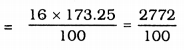= Rs. 27.72.

Question 6.
Find the radius of a sphere whose surface area is 154 cm2.
Solution:
Total surface area of a sphere= 154 cm2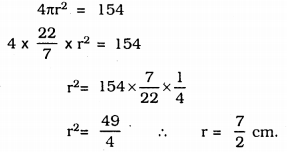∴ r = 3.5 cm

Question 7.
The diameter of the moon is approximately one-fourth of the diameter of the earth. Find the ratio of their surface areas.
Solution:
Let the diameter of earth be d’ unit
∴ Diameter of Moon = d4
radius of the earth = d2
radius of the moon = 12×d4=d8
Ratio of surface area,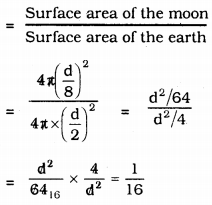= 1 : 16

Question 8.
A hemispherical bowl is made of steel, 0.25 cm thick. The inner radius of the bowl is 5 cm. Find the outer curved surface area of the bowl.
Solution:
Thickness of the hemispherical bowl = 0.25 cm.
inner radius, r = 5 cm.
∴ Outer Surfce area= 5 + 0.25 = 5.25 cm.
Outer Curved Surface area = 2πr2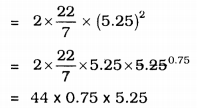= 173.25 cm2.

Question 9.
A right circular cylinder just encloses a sphere of radius r (see Fig. 13.22). Find
(i) surface area of the sphere,
(ii) curved surface area of the cylinder
(iii) ratio of the areas obtained in (i) & (ii).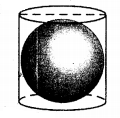Solution:
(i) If radius of a sphere is ‘r’ cm, its Surface area = 4πr2
(ii) height of cylinder, h = diameter of sphere
h = r + r
∴ h = 2r
∴ Curved surface area of cylinder = 2πrh
= 2πr × h
= 2πr × 2r
= 4πr2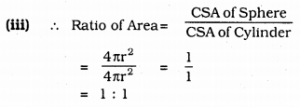= 1 : 1

All Chapter KSEEB Solutions For Class 9 Maths

—————————————————————————–

All Subject KSEEB Solutions For Class 9

*************************************************

I think you got complete solutions for this chapter. If You have any queries regarding this chapter, please comment on the below section our subject teacher will answer you. We tried our best to give complete solutions so you got good marks in your exam.

If these solutions have helped you, you can also share kseebsolutionsfor.com to your friends.

Best of Luck!!